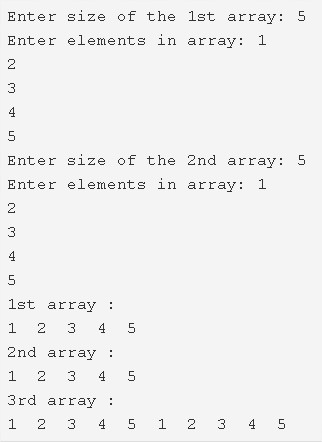# Merge Two Arrays To Third Array C Program | 4 Ways

C program to merge two arrays to the third array – In this article, we will detail in on the several ways to merge two arrays to the third array in C programming.

Suitable examples and sample programs have also been added so that you can understand the whole thing very clearly. The compiler has also been added with which you can execute it yourself for Merge Two Arrays To Third Array C Program.

The different ways used in this particular piece are as follows:

• Using Standard Method
• Using Function

An array, as we all know, is a normal arrangement of elements in any distinct order. Arrays are mostly used to represent information in a horizontal fashion.As you can see, firstly, you need to decide the size of both the arrays.

In this case, both the arrays were assigned a size of 5 each.

Then, the elements have to be added to both accordingly.

The elements added to these arrays are as follows:

Array 1: 1 2 3 4 5

Array 2: 1 2 3 4 5

After both the arrays are added, the elements will be added one after the other.

Array 3: 1 2 3 4 5 1 2 3 4 5

Thus, the methods used in this article to merge two arrays in C programming are as follows:

## Using Standard Method

1. Read the 1st array size and store it into the variable n1. Read the entered elements and store the elements into the 1st array a[] using scanf() statement, the for loop for(i=0;i<n1;i++).

2) Read the 2nd array size and store the value into the variable n2. Read the entered elements into the 2nd array b[] using scanf() statement, the for loop for(i=0;i<n2;i++).

3) Merge the two arrays a[],b[] into c[] as,

for loop iterates from i=0 to i<size of the 1st array+size of 2nd array

a) if i<size of 1st array then c[i]=a[i].Otherwise c[i]=b[i-n1].

b) After all iterations of for loop the two arraays will be merged into the array c[] and the size of c is size of 1st array+size of 2nd array.

4) Print the first array by calling print(a,n1) and print 2nd array by calling print(b,n2) and print the c[] by calling print(c,n1+n2).

Output:

### Using Function

1. The main() function calls the merge() function by passing the arrays a,b,c and 1st array size,2nd array size are as arguments.

2) The function merge() will merge the two given arrays into the 3rd array as

the for loop iterates from i=0 to i<n1+n2 where n1 is the size of the 1st array,n2 is the size of the 2nd array.

a) if i<n1 then the element of the 1st array will be copied into the array c[]. Otherwise, the element of b with the index i-n1 will be copied into the array c[]. Repeat until all iterations of for loop.

3) Print the 1st array by calling the print function as print(a,n1). Print the 2nd array by calling the print() function by passing b,n2 as arguments. Print the 3rd array by calling the print function as print(c,n1+n2).

Output:
x

## C Program To Check If Alphabet, Digit or Special Character | C Programs

C program to check for an alphabet, digit or special character – In this article, ...